# Setup for 5 Player a Game of Food Chain Magnate

Originally generated on 1/10/2019 11:41:28 PM
A direct link to this setup is http://www.VentersConsulting.com/BoardgameHelpers/FoodChainMagnateGenerator.aspx?LoadGame=\$2a\$04\$hrLhqVtMFG15oUTBdN.HKO

## Player Setup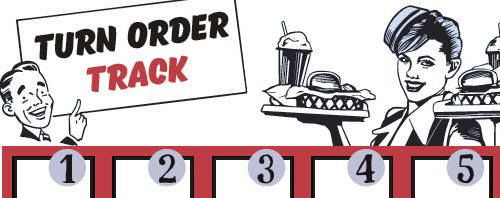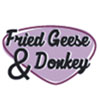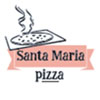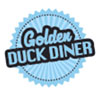## Other Setup

Bank starts with \$250.00
Number of 1x Employee cards used = 3

## Map (The Way Out Suburbs)

Click here to view Map Tile Lettering Key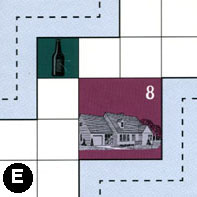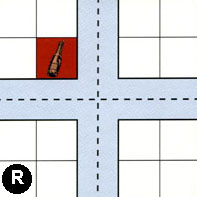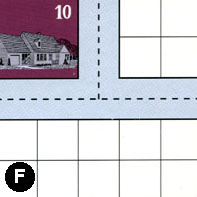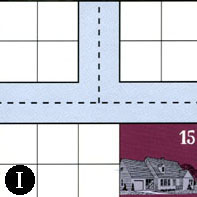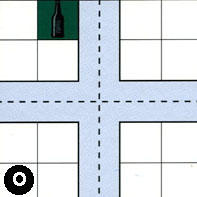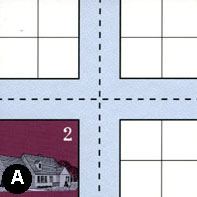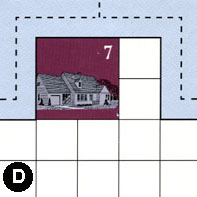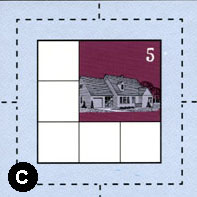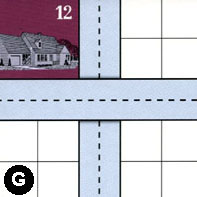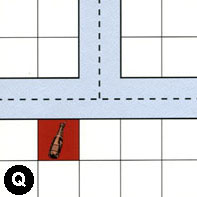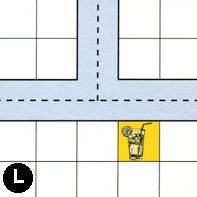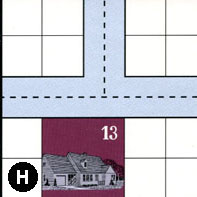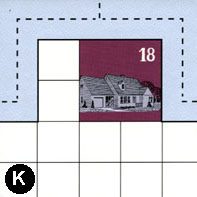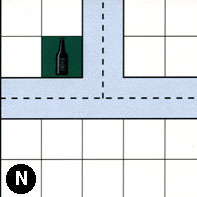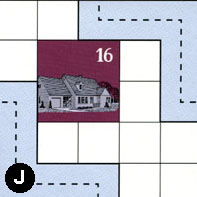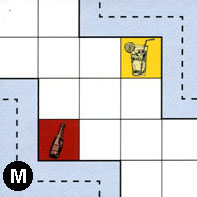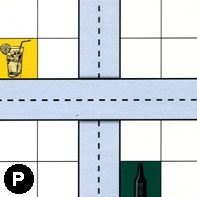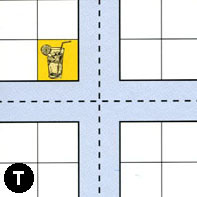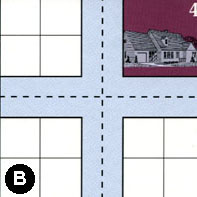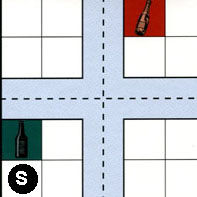### Map Stats

Map Option = The Way Out Suburbs
Total Number of Tiles = 20
Total Number of Starting Houses = 11
Total Number of Beer Spots = 5
Total Number of Soda Spots = 4
Total Number of Lemonade Spots = 4
Total Number of Drink Spots = 13
Number of independent path systems = 2
Number of independent neighborhoods = 19

Path System 1
Size = Tiny
Number of Tiles = 1
Number of Paths = 1
Contains Loop(s) = No
Number of Starting Houses = 1
Starting Houses Pct of Map (%) = 9
Number of Beer Spots = 0
Number of Soda Spots = 0
Number of Lemonade Spots = 0
Number of Drink Spots = 0
Drink Spots Pct of Map (%) = 0
Tile Ids = E
Paths = EES What do these mean?
Starting House Ids = 8
Path System 2
Size = Large
Number of Tiles = 20
Number of Paths = 75
Contains Loop(s) = No
Number of Starting Houses = 11
Starting Houses Pct of Map (%) = 100
Number of Beer Spots = 5
Number of Soda Spots = 4
Number of Lemonade Spots = 4
Number of Drink Spots = 13
Drink Spots Pct of Map (%) = 100
Tile Ids = A, B, C, D, E, F, G, H, I, J, K, L, M, N, O, P, Q, R, S, T
Paths = RWE, DWN, CWS, FWN, RWS, DWE, HWE, PWE, HWN, LWN, MWS, LNE, LWE, HNE, DNE, CNS, GWE, QWN, JWS, QWE, OWN, OWE, IWN, GNS, NWE, BWN, SWN, JEN, SNE, SWE, BNE, BWE, NWN, KWE, KWN, CWE, FWE, FNE, RNS, EWN, AWN, ANE, AWE, AWS, ANS, AES, RWN, RNE, RES, CWN, CNE, KNE, NNE, CES, BWS, TWE, PNS, MEN, TWN, TNE, BNS, SWS, SNS, SES, BES, TES, TWS, TNS, INE, IWE, ONE, QNE, ONS, OWS, OES What do these mean?
Starting House Ids = 0, 2, 4, 5, 7, 10, 12, 13, 15, 16, 18

Neighborhood 1
Total Size = Medium
Number of Total Spaces = 23
Number of Empty Spaces (for new houses & gardens) = 14
Number of Beer Spots = 1
Number of Soda Spots = 0
Number of Lemonade Spots = 0
Number of Drink Spots = 1
Number of Starting Houses = 2
Starting Houses Pct of Map (%) = 18
Tile Ids = A, E, R
Starting House Ids = 2, 8
Neighborhood 2
Total Size = Medium
Number of Total Spaces = 8
Number of Empty Spaces (for new houses & gardens) = 8
Number of Beer Spots = 0
Number of Soda Spots = 0
Number of Lemonade Spots = 0
Number of Drink Spots = 0
Number of Starting Houses = 0
Starting Houses Pct of Map (%) = 0
Tile Ids = F, R
Neighborhood 3
Total Size = Large
Number of Total Spaces = 32
Number of Empty Spaces (for new houses & gardens) = 24
Number of Beer Spots = 0
Number of Soda Spots = 0
Number of Lemonade Spots = 0
Number of Drink Spots = 0
Number of Starting Houses = 2
Starting Houses Pct of Map (%) = 18
Tile Ids = F, G, I, O
Starting House Ids = 12, 15
Neighborhood 4
Total Size = Small
Number of Total Spaces = 4
Number of Empty Spaces (for new houses & gardens) = 4
Number of Beer Spots = 0
Number of Soda Spots = 0
Number of Lemonade Spots = 0
Number of Drink Spots = 0
Number of Starting Houses = 0
Starting Houses Pct of Map (%) = 0
Tile Ids = O
Neighborhood 5
Total Size = Large
Number of Total Spaces = 46
Number of Empty Spaces (for new houses & gardens) = 40
Number of Beer Spots = 0
Number of Soda Spots = 1
Number of Lemonade Spots = 1
Number of Drink Spots = 2
Number of Starting Houses = 1
Starting Houses Pct of Map (%) = 9
Tile Ids = A, D, H, L, R
Starting House Ids = 7
Neighborhood 6
Total Size = Medium
Number of Total Spaces = 8
Number of Empty Spaces (for new houses & gardens) = 4
Number of Beer Spots = 0
Number of Soda Spots = 0
Number of Lemonade Spots = 0
Number of Drink Spots = 0
Number of Starting Houses = 1
Starting Houses Pct of Map (%) = 9
Tile Ids = F, R
Starting House Ids = 10
Neighborhood 7
Total Size = Medium
Number of Total Spaces = 16
Number of Empty Spaces (for new houses & gardens) = 16
Number of Beer Spots = 0
Number of Soda Spots = 0
Number of Lemonade Spots = 0
Number of Drink Spots = 0
Number of Starting Houses = 0
Starting Houses Pct of Map (%) = 0
Tile Ids = G, I, O, Q
Neighborhood 8
Total Size = Large
Number of Total Spaces = 55
Number of Empty Spaces (for new houses & gardens) = 49
Number of Beer Spots = 1
Number of Soda Spots = 1
Number of Lemonade Spots = 0
Number of Drink Spots = 2
Number of Starting Houses = 1
Starting Houses Pct of Map (%) = 9
Tile Ids = B, G, J, N, O, Q, S
Starting House Ids = 16
Neighborhood 9
Total Size = Medium
Number of Total Spaces = 9
Number of Empty Spaces (for new houses & gardens) = 5
Number of Beer Spots = 0
Number of Soda Spots = 0
Number of Lemonade Spots = 0
Number of Drink Spots = 0
Number of Starting Houses = 1
Starting Houses Pct of Map (%) = 9
Tile Ids = C
Starting House Ids = 5
Neighborhood 10
Total Size = Medium
Number of Total Spaces = 8
Number of Empty Spaces (for new houses & gardens) = 8
Number of Beer Spots = 0
Number of Soda Spots = 0
Number of Lemonade Spots = 0
Number of Drink Spots = 0
Number of Starting Houses = 0
Starting Houses Pct of Map (%) = 0
Tile Ids = G, N
Neighborhood 11
Total Size = Large
Number of Total Spaces = 46
Number of Empty Spaces (for new houses & gardens) = 35
Number of Beer Spots = 2
Number of Soda Spots = 0
Number of Lemonade Spots = 1
Number of Drink Spots = 3
Number of Starting Houses = 2
Starting Houses Pct of Map (%) = 18
Tile Ids = B, H, K, N, P, T
Starting House Ids = 13, 18
Neighborhood 12
Total Size = Small
Number of Total Spaces = 4
Number of Empty Spaces (for new houses & gardens) = 4
Number of Beer Spots = 0
Number of Soda Spots = 0
Number of Lemonade Spots = 0
Number of Drink Spots = 0
Number of Starting Houses = 0
Starting Houses Pct of Map (%) = 0
Tile Ids = L
Neighborhood 13
Total Size = Large
Number of Total Spaces = 27
Number of Empty Spaces (for new houses & gardens) = 25
Number of Beer Spots = 0
Number of Soda Spots = 1
Number of Lemonade Spots = 1
Number of Drink Spots = 2
Number of Starting Houses = 0
Starting Houses Pct of Map (%) = 0
Tile Ids = H, L, M, P
Neighborhood 14
Total Size = Small
Number of Total Spaces = 4
Number of Empty Spaces (for new houses & gardens) = 3
Number of Beer Spots = 0
Number of Soda Spots = 1
Number of Lemonade Spots = 0
Number of Drink Spots = 1
Number of Starting Houses = 0
Starting Houses Pct of Map (%) = 0
Tile Ids = S
Neighborhood 15
Total Size = Small
Number of Total Spaces = 4
Number of Empty Spaces (for new houses & gardens) = 3
Number of Beer Spots = 0
Number of Soda Spots = 0
Number of Lemonade Spots = 1
Number of Drink Spots = 1
Number of Starting Houses = 0
Starting Houses Pct of Map (%) = 0
Tile Ids = P
Neighborhood 16
Total Size = Medium
Number of Total Spaces = 8
Number of Empty Spaces (for new houses & gardens) = 8
Number of Beer Spots = 0
Number of Soda Spots = 0
Number of Lemonade Spots = 0
Number of Drink Spots = 0
Number of Starting Houses = 0
Starting Houses Pct of Map (%) = 0
Tile Ids = P, T
Neighborhood 17
Total Size = Medium
Number of Total Spaces = 8
Number of Empty Spaces (for new houses & gardens) = 8
Number of Beer Spots = 0
Number of Soda Spots = 0
Number of Lemonade Spots = 0
Number of Drink Spots = 0
Number of Starting Houses = 0
Starting Houses Pct of Map (%) = 0
Tile Ids = B, T
Neighborhood 18
Total Size = Medium
Number of Total Spaces = 8
Number of Empty Spaces (for new houses & gardens) = 3
Number of Beer Spots = 1
Number of Soda Spots = 0
Number of Lemonade Spots = 0
Number of Drink Spots = 1
Number of Starting Houses = 1
Starting Houses Pct of Map (%) = 9
Tile Ids = B, S
Starting House Ids = 4
Neighborhood 19
Total Size = Small
Number of Total Spaces = 4
Number of Empty Spaces (for new houses & gardens) = 4
Number of Beer Spots = 0
Number of Soda Spots = 0
Number of Lemonade Spots = 0
Number of Drink Spots = 0
Number of Starting Houses = 0
Starting Houses Pct of Map (%) = 0
Tile Ids = S

v8.3.2